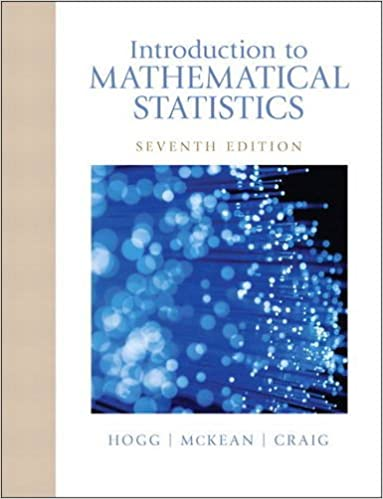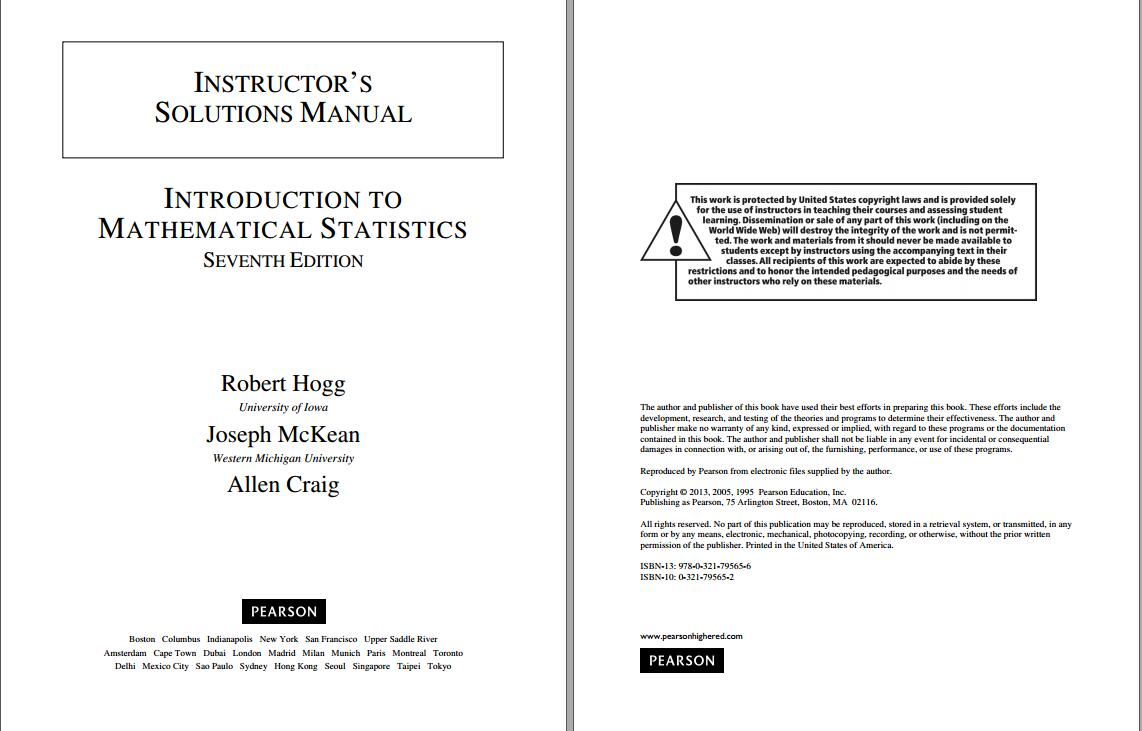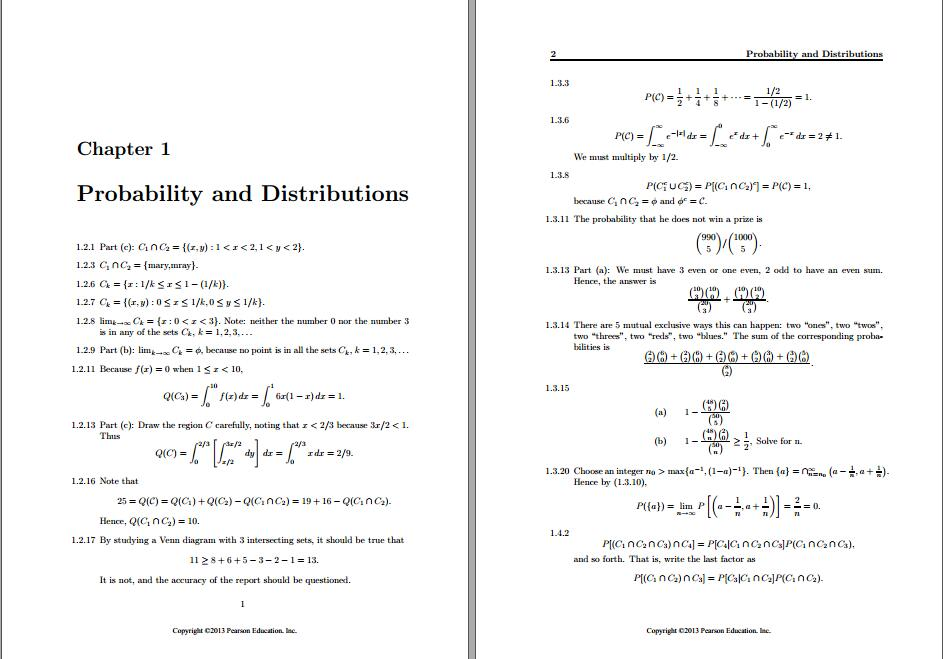# Solution manual for Introduction to Mathematical Statistics 7th Edition by Robert Hogg

name：Solution manual for Introduction to Mathematical Statistics 7th Edition by Robert Hogg
Edition： 7th Edition
author：by Robert Hogg, Joseph McKean, Allen Craig
ISBN：ISBN-13: 9780321795434
ISBN-10: 0321795431
type：solution manual
format：word/zip
All chapter include

Introduction to Mathematical Statistics, Seventh Edition, offers a proven approach designed to provide you with an excellent foundation in mathematical statistics. Ample examples and exercises throughout the text illustrate concepts to help you gain a solid understanding of the material.

##### Cover + PreviewSolution manual for Introduction to Mathematical Statistics 7th Edition by Robert HoggSolution manual for Introduction to Mathematical Statistics 7th Edition by Robert HoggSolution manual for Introduction to Mathematical Statistics 7th Edition by Robert Hogg

##### Free Sample
• smch1 • 689 kB • 113 click
Price：59 points    [1\$ USD=5points]
VIP Price：20% off